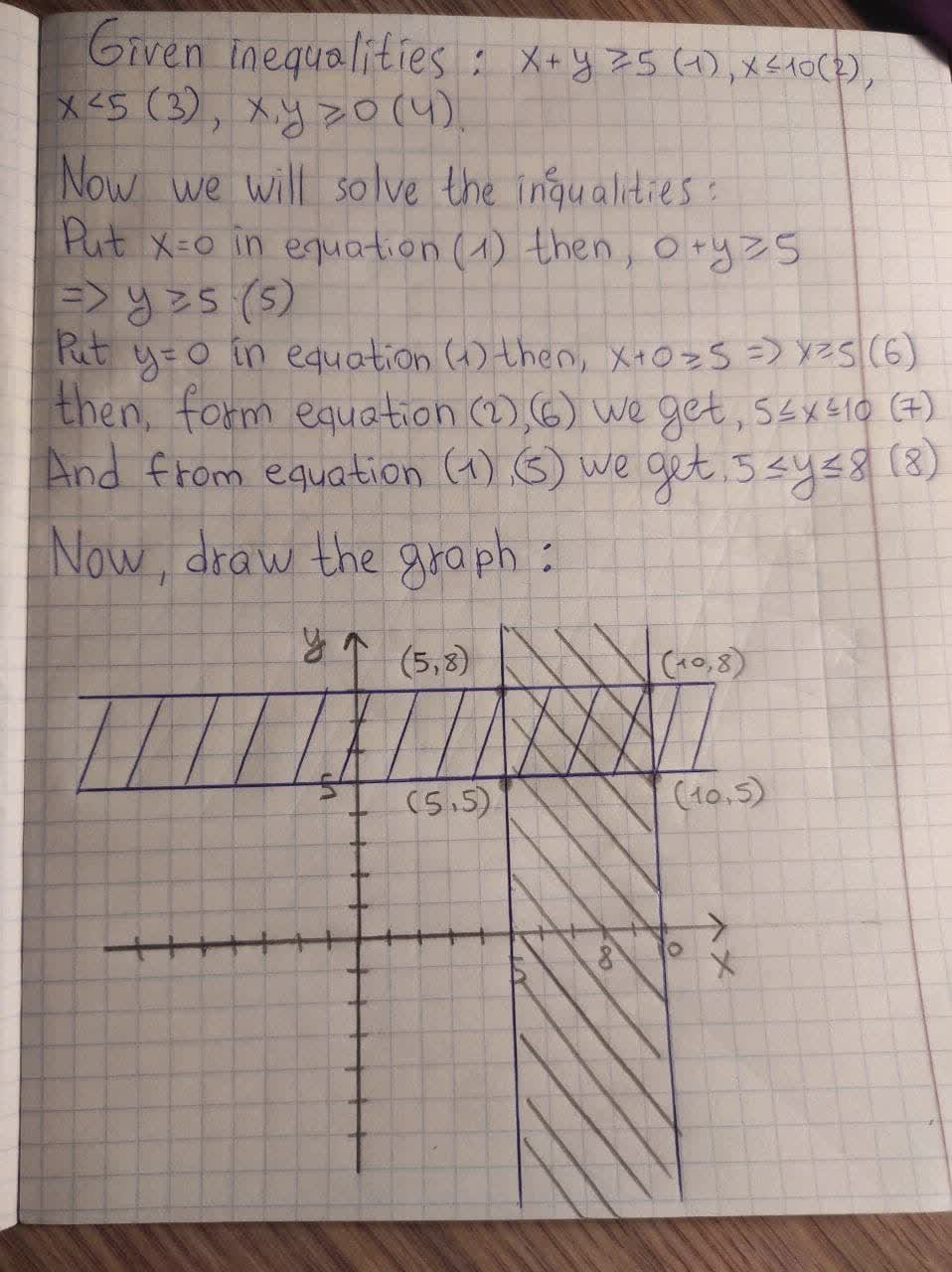# Sketch the region that corresponds to the given inequalities, say whether the region is bounded or unbounded, and find the coordinates of all corner points (if any). x + y >= 5 (1) x <= 10 (2) y <= 5 (3) x,y >= 0 (4)boitshupoO 2020-12-07 Answered
Sketch the region that corresponds to the given inequalities, say whether the region is bounded or unbounded, and find the coordinates of all corner points (if any).
$x+y\ge 5\left(1\right)$
$x\le 10\left(2\right)$
$y\le 5\left(3\right)$
$x,y\ge 0\left(4\right)$
You can still ask an expert for help

## Want to know more about Inequalities systems and graphs?

• Questions are typically answered in as fast as 30 minutes

Solve your problem for the price of one coffee

• Math expert for every subject
• Pay only if we can solve itavortarF
Now we will solve the inequalities as,
Put x=0 in eqution (1) then, $0+y\ge 5$
rArr $y\ge 5$ (5)
Again, Put y=0 in equation (1) then, $x+0\ge 5$
rArr $x\ge 5$ (6)
Then, from equation (2), (6) we get,
$5\le x\le 10$ (7)
And from equation (1), (5) we get,
$5\le y\le 8$ (8)
Now, draw the graph